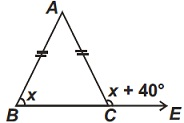# User Forum

Subject :IMO    Class : Class 7

In the following figure, if AB = AC, then x isA 80°
B 70°
C 60°
D 110°

## Ans 1:

Class : Class 9
Angle BAC = x + 40 - x => 40 As triangle ABC is an isosceles triangle with AB = AC, angle acb = angle abc = x Therefore, as angle abc + angle acb + angle bac = 180 then, x + x + 40 = 180 =>2x = 180 - 40 =>2x = 140 =>x = 70

Class : Class 9
d

Class : Class 7
D